## A student is going to take a five-question, multiple-choice quiz. Each question has exact four choices for the answer, but only one of which

Question

A student is going to take a five-question, multiple-choice quiz. Each question has exact four choices for the answer, but only one of which is correct. The student forgets to study for the quiz and plans to randomly guess on each question. Let X be the number of correct answers he will obtains. Answer the following questions. Find the probability that he obtains at most one correct answer

in progress 0
5 months 2021-08-27T04:35:06+00:00 1 Answers 6 views 0

0.6328 = 63.28% probability that he obtains at most one correct answer

Step-by-step explanation:

For each question, there are only two possible outcomes. Either he obtains the correct answer, or he does not. The probability of obtaining the correct answer on a question is independent of any other questions, which means that the binomial probability distribution is used to solve this question.

Binomial probability distribution

The binomial probability is the probability of exactly x successes on n repeated trials, and X can only have two outcomes.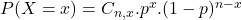In which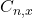is the number of different combinations of x objects from a set of n elements, given by the following formula.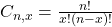And p is the probability of X happening.

Five-question

This means that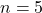Each question has exact four choices for the answer, but only one of which is correct.

This means that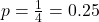Find the probability that he obtains at most one correct answer

This is:In which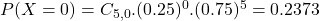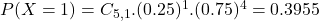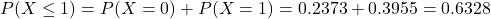0.6328 = 63.28% probability that he obtains at most one correct answer